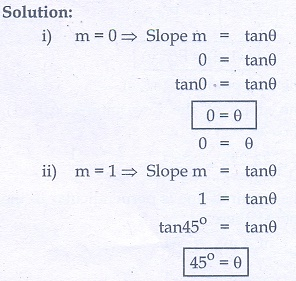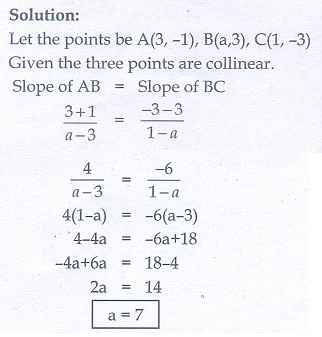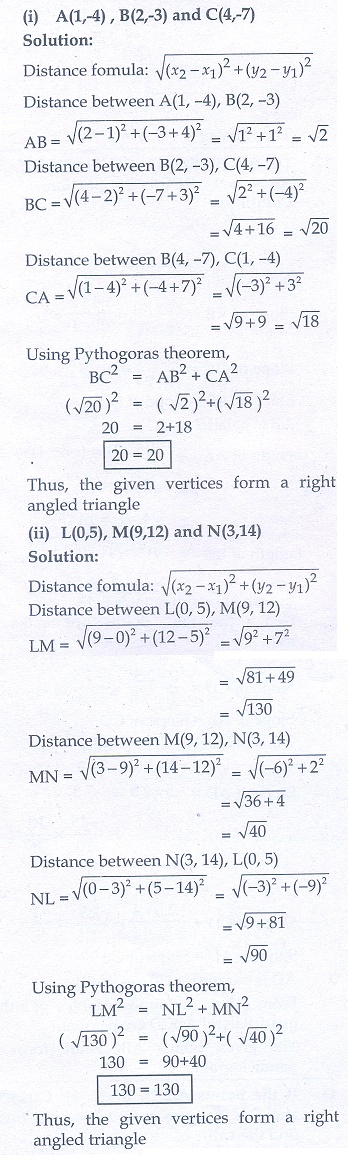Home | | Maths 10th Std | Exercise 5.2: Inclination of a line

# Exercise 5.2: Inclination of a line

Maths Book back answers and solution for Exercise questions - Mathematics : Coordinate Geometry: Inclination of a line: Exercise Problem Questions with Answer

Exercise 5.2

1. What is the slope of a line whose inclination with positive direction of x -axis is

(i) 90°

(ii) 0°2. What is the inclination of a line whose slope is

(i) 0

(ii) 13. Find the slope of a line joining the points

(i) (5, √5) with the origin

(ii) (sin θ, - cos θ) and (-sin θ, cos θ)4. What is the slope of a line perpendicular to the line joining A(5, 1) and P where P is the mid-point of the segment joining (4, 2) and (-6, 4).5. Show that the given points are collinear: (- 3, - 4) , (7, 2) and (12, 5)6. If the three points (3, -1) , (a , 3) and (1, - 3) are collinear, find the value of a.7. The line through the points (-2,a) and (9, 3) has slope – 1/2. Find the value of a.8. The line through the points (-2, 6) and (4,8) is perpendicular to the line through the points (8,12) and (x , 24) . Find the value of x.9. Show that the given points form a right angled triangle and check whether they satisfies pythagoras theorem

(i) A(1, - 4) , B(2, - 3) and C(4, - 7)

(ii) L(0, 5) , M(9, 12) and N(3, 14)10. Show that the given points form a parallelogram:

A(2.5, 3.5) , B(10, - 4) , C(2.5, - 2.5) and D(-5, 5)11. If the points A(2,2), B(–2, –3), C(1, –3) and D(xy) form a parallelogram then find the value of x and y.12. Let A(3, - 4) , B(9, - 4) , C(5, - 7) and D(7, - 7) . Show that ABCD is a trapezium.13. A quadrilateral has vertices at A(- 4, - 2) , B(5, -1) , C(6, 5) and D(-7, 6) . Show that the mid-points of its sides form a parallelogram.14. PQRS is a rhombus. Its diagonals PR and QS intersect at the point M and satisfy QS = 2PR. If the coordinates of S and M are (1, 1) and (2, -1)respectively, find the coordinates of P.1. (i) undefined (ii) 0

2. (i) 0° (ii) 45°

3. (i) 1/√5 (ii) -cot θ

4. 3

6. 7

7. 17/2

8. 4

9. (i) yes (ii) yes

11. 5, 2

14. (1 -3/2) or (3, -1/2)

Tags : Problem Questions with Answer, Solution | Mathematics , 10th Mathematics : UNIT 5 : Coordinate Geometry
Study Material, Lecturing Notes, Assignment, Reference, Wiki description explanation, brief detail
10th Mathematics : UNIT 5 : Coordinate Geometry : Exercise 5.2: Inclination of a line | Problem Questions with Answer, Solution | Mathematics